## Bernoulli bond percolation

The model is defined as follows. Consider the integer lattice Zd with nearest-neighbor edges. Each edge is marked open with probability p and closed with probability 1 - p, independently of each other. Here are some pictures of Bernoulli bond percolation on Z2. (The edges we keep are the open edges, and the edges we remove are the closed edges.)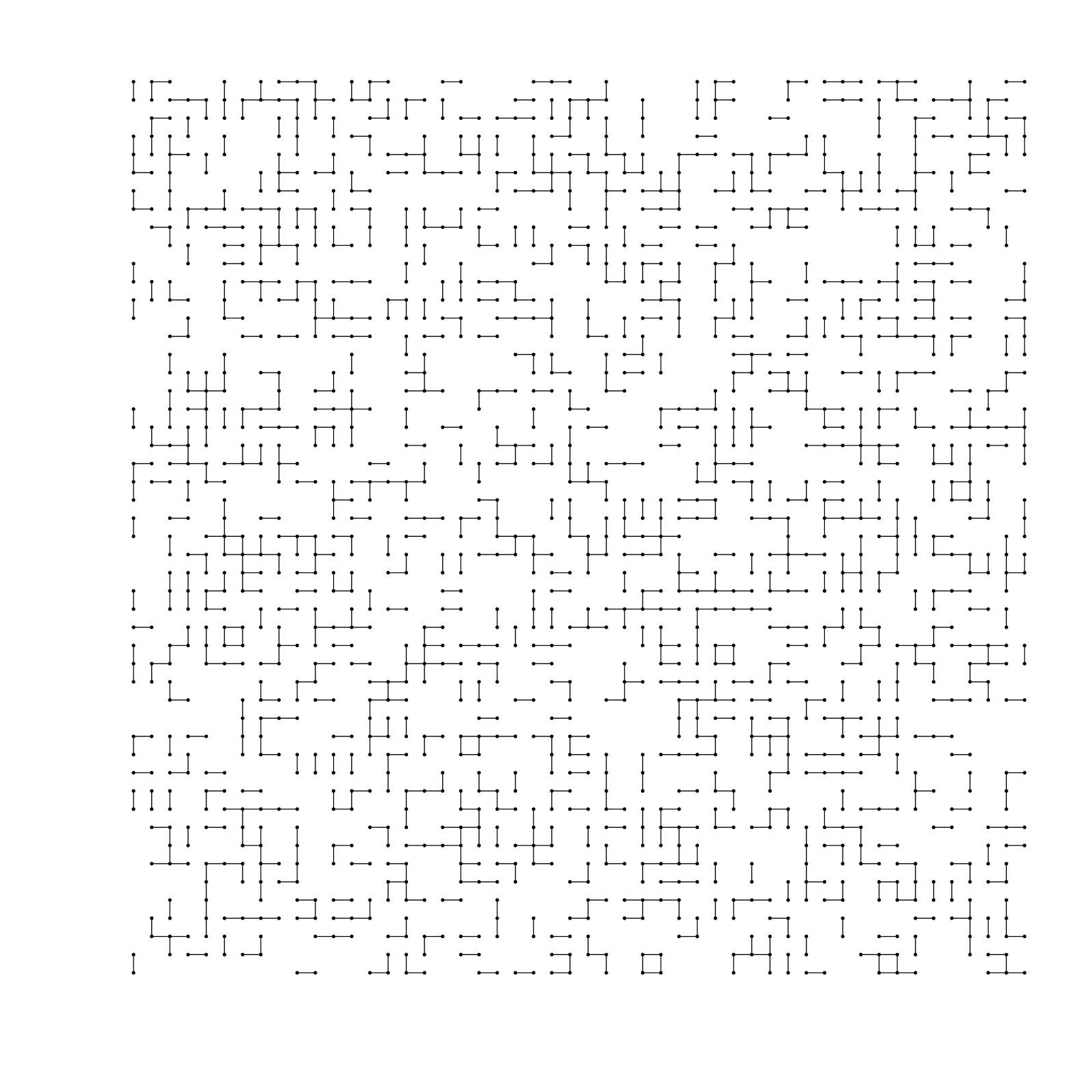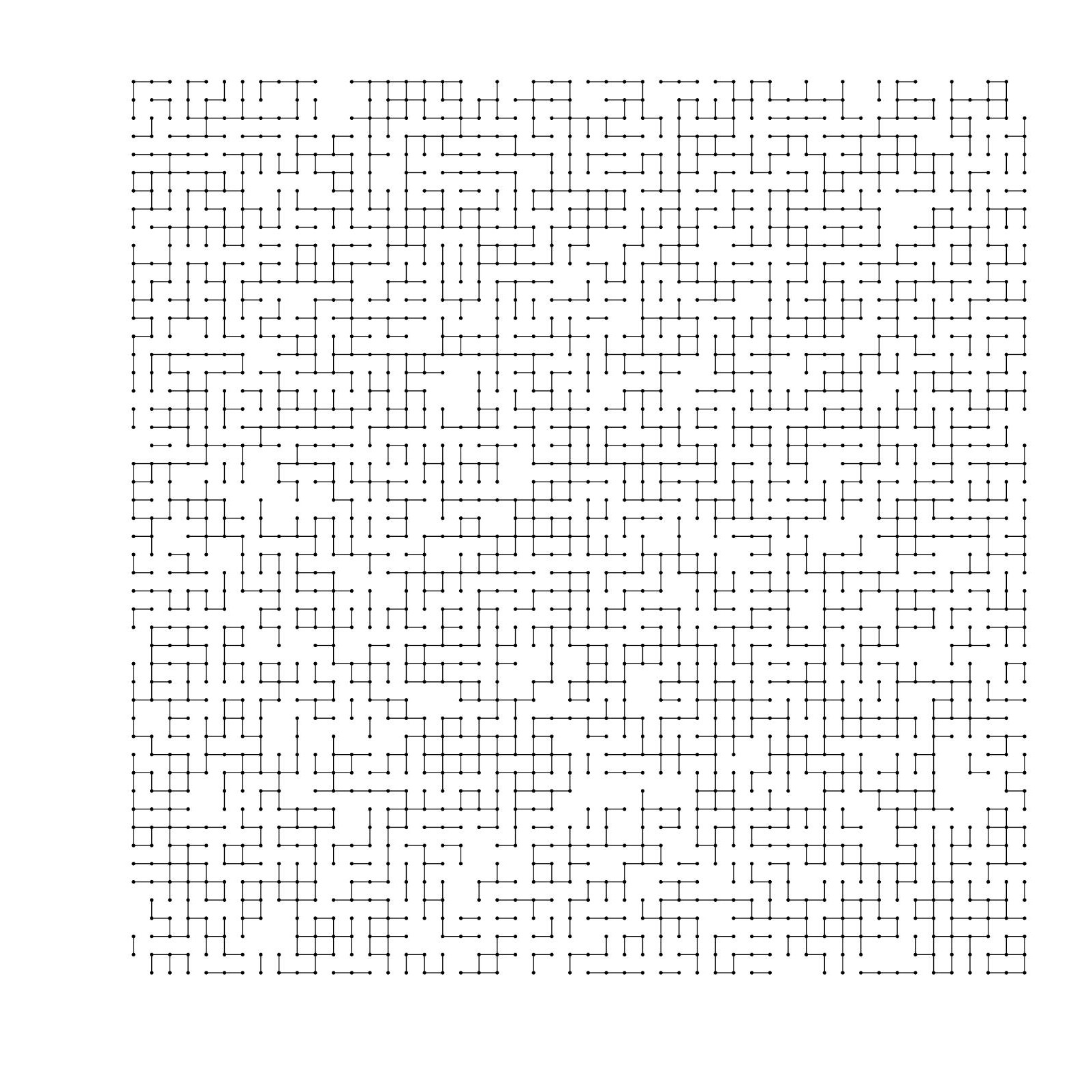p = 0.25 p = 0.5 p = 0.75

When p is sufficiently large, almost surely, there exists an infinite connected component of open edges. When p is small, there are only finite component of open edges. There is a phase transition occurs at some critical value p = pc. When d = 2, this critical value pc = 0.5.

## Bernoulli site percolation

Site percolation is very similar to bond percolation. The only difference is that vertices are kept or removed instead of edges. There is also a phase transition. When d = 2, pc is approximately 0.59.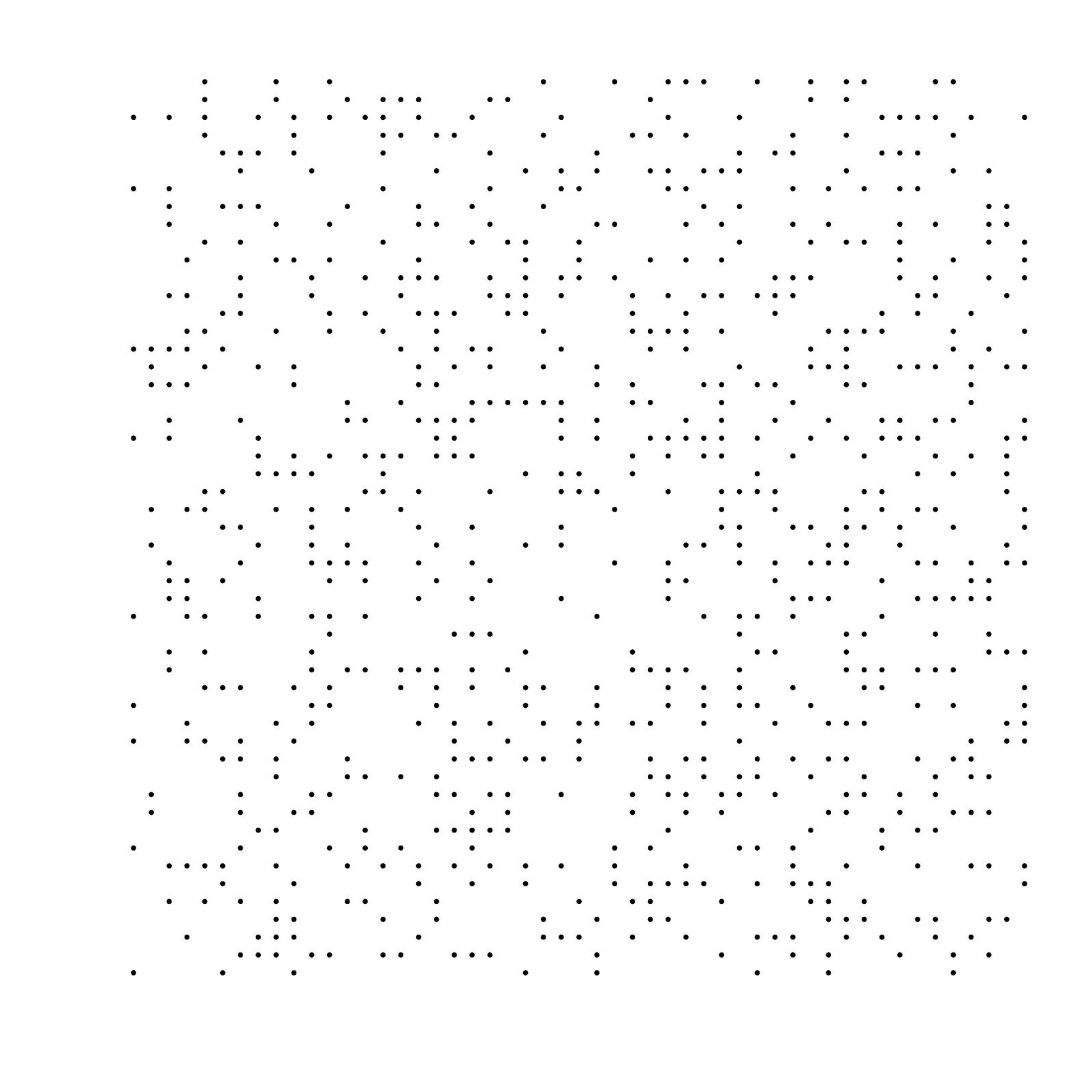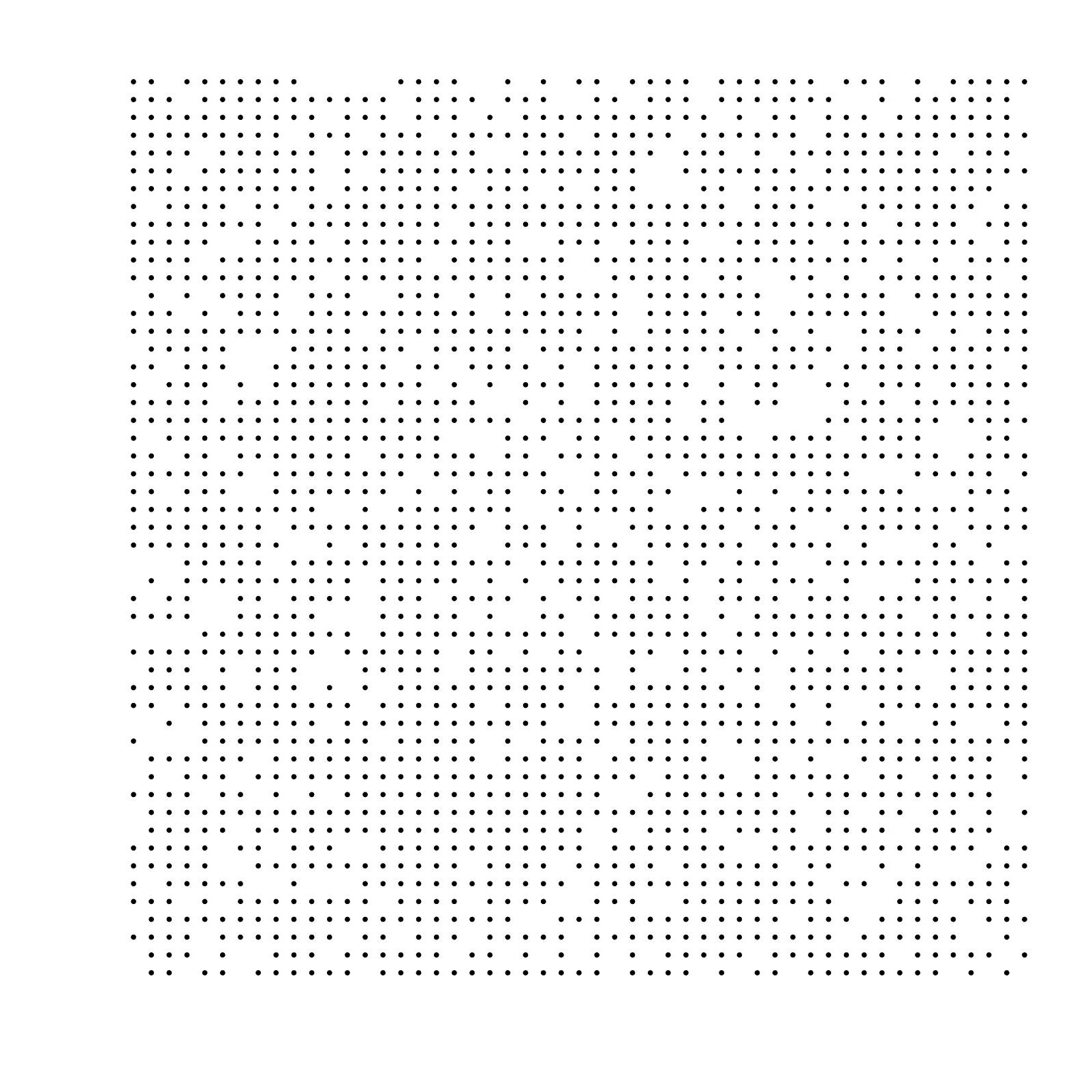p = 0.3 p = 0.59 p = 0.8

## First-passage percolation

Again consider Zd with nearest-neighbor edges. Each edge e is assigned a nonnegative random passage time te. Assume that the random variables (te) are independent and identically distributed. The passage time along a path γ is defined as T(γ) = ∑eγ te. For any two points x, y in Zd, we define the first-passage time from x to y by T(x, y) = inf{T(γ) : γ is a path from x to y}. This T defines a (pseudo)metric on Zd. How does a metric ball with large radius look like?Above is a simulation of a metric ball with large radius on Z2, with te exponentially distributed. It is known that the ball, divided by its radius, will converge (in certain sense) to a nonrandom, compact, convex set B with nonempty interior as the radius goes to infinity. It is conjectured that B is not the Euclidean ball.

## Frozen percolation

Consider the planar lattice Z2. Fix a large integer N. (Volume-)frozen percolation with parameter N is defined as follows. Initially, all the vertices are vacant. Each vertex will try to become occupied, but is allowed to do so only if each of its neighbor is in an occupied cluster of size no larger than N. In other words, clusters grow until their sizes become at least N. When an occupied cluster has size at least N, we say that the cluster is frozen. Here are some pictures of frozen percolation in a 100×100 box with different N: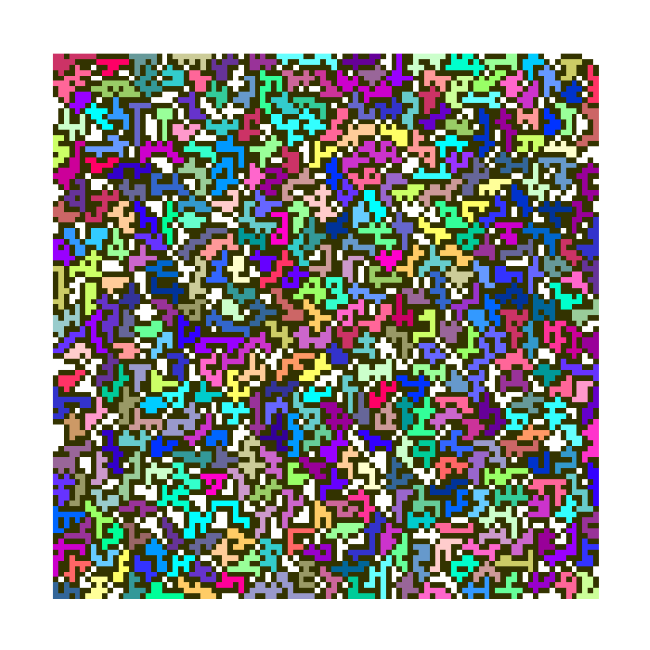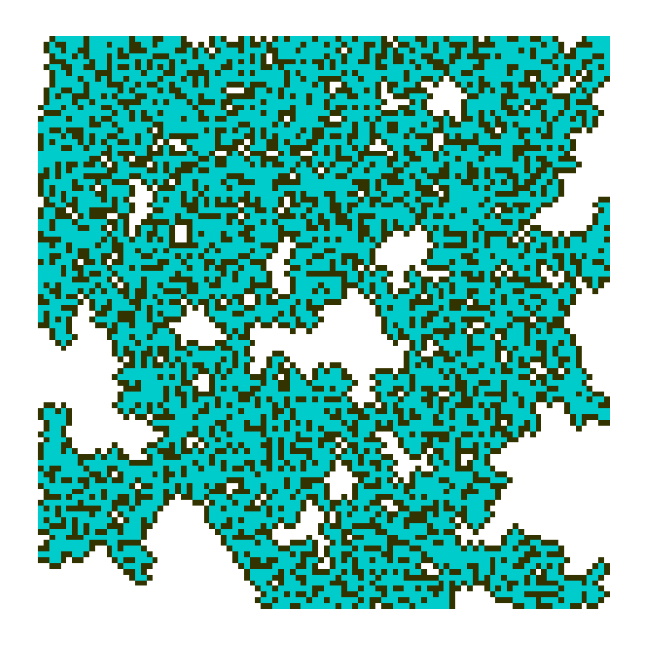N = 10 N = 100 N = 3000

Each colored region is a frozen cluster. This model exhibits the so-called "self-organized criticality": we don't need to fine tune the parameter to a precise value to see the "critical" behavior.

## Forest fire processes

There are many variants of forest fire processes (but none of them is realistic). Many of them have these two features: 1. clusters (or trees) grow in some random manner, and 2. large clusters (or forests) will be burned by some random rule. In fact, frozen percolation defined above can be seen as a forest fire prcoess, if one thinks frozen clusters as burned clusters instead.

Consider the following forest fire process: trees grow at vertices in Z2 at rate 1 according to independent Poisson processes. When there is a left-right crossing of trees, we burn the whole crossing. Trees can grow again at the burned sites. Interesting phenomenon will occur around time tc := -log(1 - pc), which is approximately 0.89. Here is a simulation: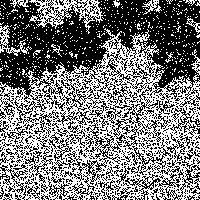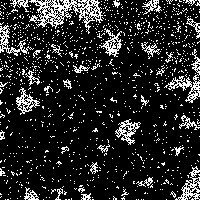t = 0.88 t = 0.89 t = 0.95 t = 0.96
The crossing burned round t = 0.89 is too "small", so there is another burning shortly after that. Here is another simulation: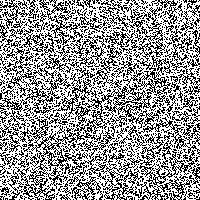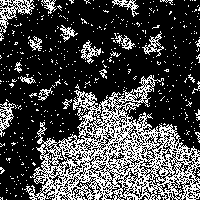t = 0.90 t = 0.91 t = 1.71 t = 1.72
This time, the burned crossing at time 0.91 is large enough, and so there is no further burning until t = 1.72 (somewhat close to twice of tc).

Now we consider another variant of forest fire processes. We will burn everything that is connected to the left boundary, and we do not allow recovery (trees cannot grow at the burned sites). Here are some pictures: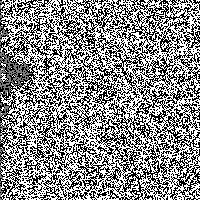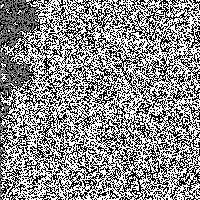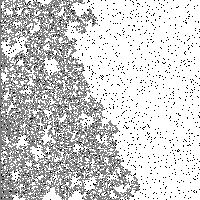t = 0.80 t = 0.84 t = 0.91 t = 3.00
Here, the gray clusters are the burned ones. Again, interesting phenomenon occurs around time tc. Another sample: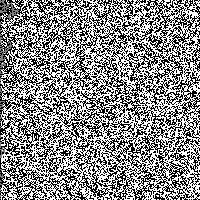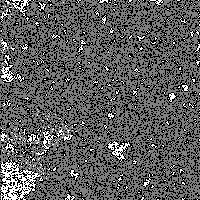t = 0.78 t = 0.79 t = 1.04 t = 1.05
In this simulation, many small clusters are burned at earlier times, which prevent burning around tc. When there is a further burning at t = 1.05, because it is far beyond tc, the cluster we burn is a "supercritical" cluster, which is relatively huge.